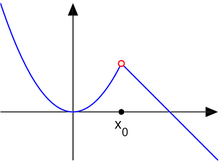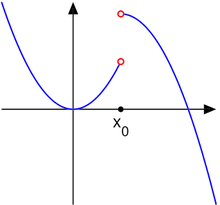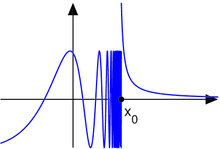# Classification of discontinuities

Classification of discontinuities

Continuous functions are of utmost importance in mathematics and applications. However, not all functions are continuous. If a function is not continuous at a point in its domain, one says that it has a discontinuity there. The set of all points of discontinuity of a function may be a discrete set, a dense set, or even the entire domain of the function.

This article describes the classification of discontinuities in the simplest case of functions of a single real variable taking real values.

## Classification of discontinuitiesThe derivative of this curve has a jump discontinuity.

Consider a real valued function ƒ of a real variable x, defined in a neighborhood of the point x0 in which ƒ is discontinuous. Then three situations may be distinguished:

1. The one-sided limit from the negative direction$L^{-}=\lim_{x\rarr x_0^{-}} f(x)$
and the one-sided limit from the positive direction$L^{+}=\lim_{x\rarr x_0^{+}} f(x)$
at x0 exist, are finite, and are equal to L = L = L + . Then, if ƒ(x0) is not equal to L, x0 is called a removable discontinuity. This discontinuity can be 'removed to make ƒ continuous at x0', or more precisely, the function$g(x) = \begin{cases}f(x) & x\ne x_0 \\ L & x = x_0\end{cases}$
is continuous at x=x0.
2. The limits L and L + exist and are finite, but not equal. Then, x0 is called a jump discontinuity or step discontinuity. For this type of discontinuity, the function ƒ may have any value in x0.
3. One or both of the limits L and L + does not exist or is infinite. Then, x0 is called an essential discontinuity, or infinite discontinuity. (This is distinct from the term essential singularity which is often used when studying functions of complex variables.)

The term removable discontinuity is sometimes incorrectly used for cases in which the limits in both directions exist and are equal, while the function is undefined at the point x0. This use is improper because continuity and discontinuity of a function are concepts defined only for points in the function's domain. Such a point not in the domain, is properly named a removable singularity.

The oscillation of a function at a point quantifies these discontinuities as follows:

• in a removable discontinuity, the distance that the value of the function is off by is the oscillation;
• in a jump discontinuity, the size of the jump is the oscillation (assuming that the value at the point lies between these limits from the two sides);
• in an essential discontinuity, oscillation measures the failure of a limit to exist.

## ExamplesThe function in example 1, a removable discontinuity

1. Consider the function$f(x)=\begin{cases}x^2 & \mbox{ for } x< 1 \\ 0 & \mbox { for } x=1 \\ 2-x& \mbox{ for } x>1\end{cases}$

Then, the point x0 = 1 is a removable discontinuity.The function in example 2, a jump discontinuity

2. Consider the function$f(x)=\begin{cases}x^2 & \mbox{ for } x< 1 \\ 0 & \mbox { for } x=1 \\ 2-(x-1)^2& \mbox{ for } x>1\end{cases}$

Then, the point x0 = 1 is a jump discontinuity.The function in example 3, an essential discontinuity

3. Consider the function$f(x)=\begin{cases}\sin\frac{5}{x-1} & \mbox{ for } x< 1 \\ 0 & \mbox { for } x=1 \\ \frac{0.1}{x-1}& \mbox{ for } x>1\end{cases}$

Then, the point x0 = 1 is an essential discontinuity (sometimes called infinite discontinuity). For it to be an essential discontinuity, it would have sufficed that only one of the two one-sided limits did not exist or were infinite. However, given this example the discontinuity is also an essential discontinuity for the extension of the function into complex variables.

## The set of discontinuities of a function

The set of points at which a function is continuous is always a Gδ set. The set of discontinuities is an Fσ set.

The set of discontinuities of a monotonic function is at most countable. This is Froda's theorem.

Thomae's function is discontinuous at every rational point, but continuous at every irrational point.

The indicator function of the rationals, also known as the Dirichlet function, is discontinuous everywhere.

Wikimedia Foundation. 2010.

### Look at other dictionaries:

• Landslide classification — There have been known various classifications of landslides and other types of mass wasting.For example, the McGraw Hill Encyclopedia of Science and Technology distinguishes the following types of landslies: *fall (by undercutting) *fall (by… …   Wikipedia

• Race (classification of humans) — Race Classification Race (classification of humans) Genetics …   Wikipedia

• Size Strength classification — is a two parameter rock classification based on the strength of intact rock and the spacing of discontinuities in the rock mass. It was developed by Louis and Franklin (1970 75). The size strength approach to rock mass characterisation has been… …   Wikipedia

• List of mathematics articles (C) — NOTOC C C closed subgroup C minimal theory C normal subgroup C number C semiring C space C symmetry C* algebra C0 semigroup CA group Cabal (set theory) Cabibbo Kobayashi Maskawa matrix Cabinet projection Cable knot Cabri Geometry Cabtaxi number… …   Wikipedia

• Continuous function — Topics in Calculus Fundamental theorem Limits of functions Continuity Mean value theorem Differential calculus  Derivative Change of variables Implicit differentiation Taylor s theorem Related rates …   Wikipedia

• Oscillation (mathematics) — For other uses, see Oscillation (differential equation). Oscillation of a sequence (shown in blue) is the difference between the limit superior and limit inferior of the sequence. In mathematics, oscillation is the behaviour of a sequence of real …   Wikipedia

• Dark energy — Not to be confused with dark matter, dark fluid, or dark flow. Physical cosmology …   Wikipedia

• Discontinuity (geotechnical engineering) — A discontinuity in geotechnical engineering (in geotechnical literature often denoted by joint) is a plane or surface that marks a change in physical or chemical characteristics in a soil or rock mass. A discontinuity can be, for example, a… …   Wikipedia

• Earth Sciences — ▪ 2009 Introduction Geology and Geochemistry       The theme of the 33rd International Geological Congress, which was held in Norway in August 2008, was “Earth System Science: Foundation for Sustainable Development.” It was attended by nearly… …   Universalium

• Mining rock mass rating — Laubscher developed the Mining Rock Mass Rating (MRMR) system by modifying the Rock Mass Rating (RMR) system of Bieniawsk …   Wikipedia